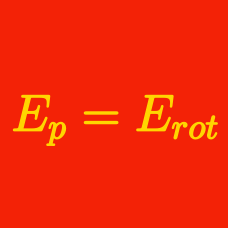Classical Mechanics

# Conservative Forces - Problem Solving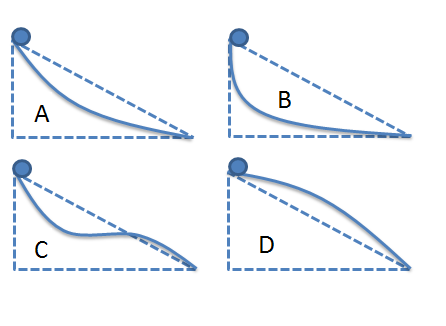Four identical balls roll down from the tops of four different triangles to the ground, along the solid lines shown in the figures above. If the heights of all four triangles are equal, to which of the four balls does gravity deliver the most work?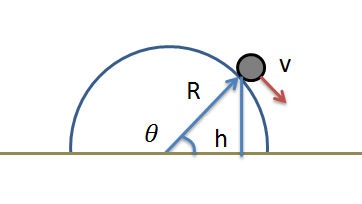A ball of mass $m = 5 \text{ kg }$ slides down from the top of a hemisphere of radius $R = 6 \text{ m }.$ The speed of the ball when it loses contact with the hemisphere can be expressed as $v = \sqrt{\frac{a}{b}} \text{ m/s},$ where $a$ and $b$ are coprime positive integers. Find the value of $a+b.$

The gravitational acceleration is $g= 10 \text{ m/s}^2.$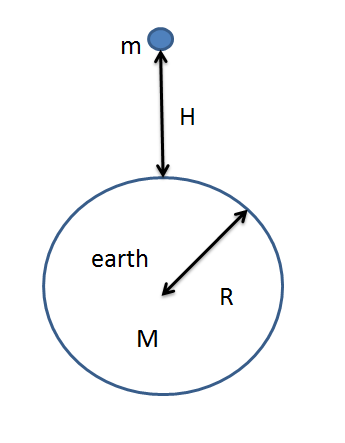A ball of mass $m = 7 \text{ kg}$ falls towards the ground from a height of $H = 8000 \text{ km} .$ When the ball's height is $h = 3000 \text{ km} ,$ its speed can be expressed as $v = \sqrt{\frac{GM\times a}{b}} \text{ km/s},$ where $a$ and $b$ are coprime positive integers. If the air resistance is negligible, what is the value of $a+b?$

$G$ is gravitational constant and $M$ is the mass of the earth. The radius of the earth is $R = 6000 \text{ km} .$A ball of mass $m = 5 \text{ kg }$ slides down from the top of a frictionless hemisphere of radius $R = 12 \text{ m} .$ At what height $h$ does the ball lose contact with the hemisphere?

The gravitational acceleration is $g= 10 \text{ m/s}^2.$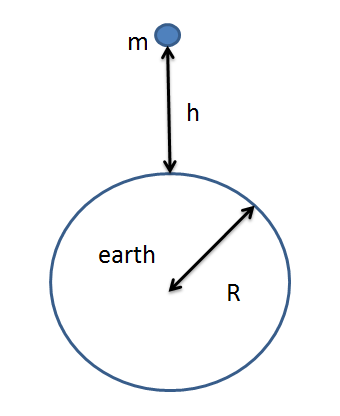A ball of mass $m = 5 \text{ kg}$ falls down towards the ground from a height of $h = 3000 \text{ km}.$ The speed with which the ball strikes the ground is $v = \sqrt{\frac{GM\times{a}}{b}} \text{ km/s},$ where $a$ and $b$ are coprime positive integers. If the air resistance is negligible, what is the value of $a+b?$

$G$ is the gravitational constant and $M$ is the mass of the earth. The radius of the earth is $R = 6000 \text{ km} .$

×